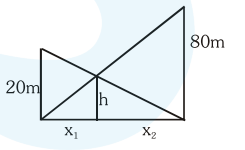# Two vertical poles of heights,`
Question:

Two vertical poles of heights, $20 \mathrm{~m}$ and $80 \mathrm{~m}$ stand a part on a horizontal plane. The height (in meters) of the point of intersection of the lines joining the top of each pole to the foot of the other, from this horizontal plane is :

1. 12

2. 15

3. 16

4. 18

Correct Option: , 3

Solution:by similar triangle

$\frac{\mathrm{h}}{\mathrm{x}_{1}}=\frac{80}{\mathrm{x}_{1}+\mathrm{x}_{2}}$ ............(1)

by $\frac{h}{x_{2}}=\frac{20}{x_{1}+x_{2}}$ .............(2)

by (1) and (2)

$\frac{x_{2}}{x_{1}}=4$ or $x_{2}=4 x_{1}$

$\Rightarrow \frac{h}{x_{1}}=\frac{80}{5 x_{1}}$

or $h=16 m$